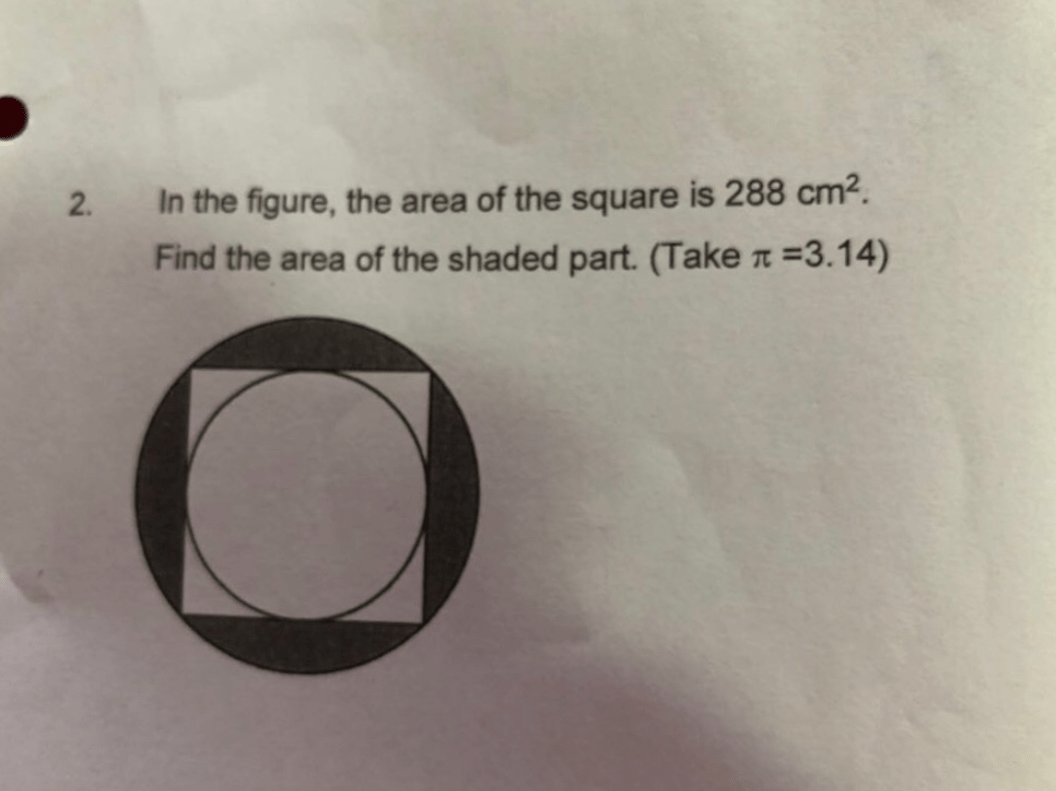# QuestionPlease help. Can we have exact answer without rounding to nearest decimal places?

1 Answer

# Answer

288/2 = 144 (area of 1/2 square) = (1/2) x 2r x r = r^2
r = sq rt 144 = 12
area of blgger/outer circle = 3.14 x 12 x 12 = 452.16
area of shaded part = 452.16 – 288 = 164.16

Ans : 164.16 sq cm.

1 Reply 0 Likes

Hi, may I know why r = sq rt 144 = 12?

1 Reply 0 Likes

288/2 = 144 (area of 1/2 square) =  (1/2) x 2r x r = r^2 (Note : A triangle, area of which is (1/2) x base, 2r x height, r  is formed when you draw a diagonal in the square)
r = sq rt 144 = 12

0 Replies 1 Like